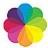# Ratio & ProportionQuantitative Aptitude Questions and Answers section on “Ratio & Proportion” with solution and explanation for competitive examinations such as CAT, MBA, SSC, Bank PO, Bank Clerical and other examinations.

# If two items of A is equal to three times of B and also equal to four times of C, then A : B : C is :

If two items of A is equal to three times of B and also equal to four times of C, then A : B : C is : [A]2 : 3 : 5 [B]3 : 4 : 2 [C]4 : 6 : 3 [D]6 : 4 : 3 Show Answer 6 : 4 : 3 ..